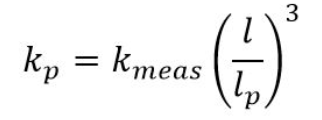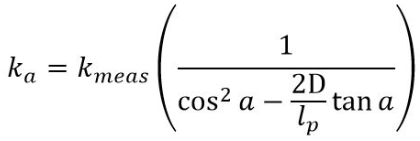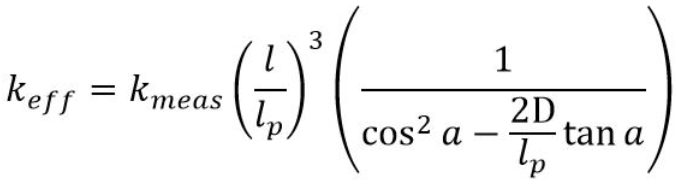# Why is the Effective Spring Constant important?

#### What is the spring constant?

As the name implies the spring constant k [N/m] describes how much force [N] is needed to squeeze a spring system by a certain distance [m].

In case of the FluidFM probes, the spring constant relates the probe deflection in [m] with the corresponding force [N] that acts on the probe in order to achieve this deflection.

#### Measuring the spring constant

In the FluidFM system, the most common method to measure the spring constant is the Sader method. It relies on the probe dimensions and its first resonance peak. It is important to mention that this method gives the spring constant for a force acting perpendicular to the very end of the probe (more details can be found in Dörig, 2013).

#### The effective spring constant

However, the probe is at an angle to the surface and interacts at the pyramid, a few microns from its edge. The force needed to displace the cantilever by 100 nm increases as the place of interactions get further away from the cantilever edge. For FluidFM nanopipettes, the place where the force is acting is the pyramid. In this case, the spring constant at the pyramid can be calculated as following  :where kp is the spring constant at the pyramid, kmeas the measured spring constant according to Sader, l the length of the cantilever (typically 200 µm) and lp the length of the cantilever until the pyramid (typically 190 µm), or the length until the opening in case of the micro pipettes (193 µm) (Sader, 1995).

As seen through beam mechanics, pressing an inclined cantilever against a surface increases the required force to bend it. This is the case for FluidFM systems, where the cantilever comes with an angle of 11° towards the surface. In this particular case, the spring constant due to this angle will follow the next equation:where ka is the spring constant due to the angle a and the pyramid height D (7 µm). For colloidal probes, the colloid radius has to be added to(Heim et al. 2004).

Finally, the effective spring constant, which takes into account the angle to the surface and the interaction with the pyramid (instead of the very end of the probe):#### Typical correction factors

For typical FluidFM probes, the total correction factor for the spring constant is as follows:

 FluidFM Probe Type keff/kmeas FluidFM Nanopipette & Nanosyringe 1.229 FluidFM Micropipette 1.155 FluidFM Micropipette with 10 µm colloid 1.167

#### References

J. E. Sader. Method for the calibration of atomic force microscope cantilevers. Review of Scientific Instruments (1995), 66 (7), 3789.

L.-O. Heim, M. Kappl, H.-J. Butt. Tilt of Atomic Force Microscope Cantilevers: Effect on Spring Constant and Adhesion Measurements. Langmuir (2014), 20 (7), 2760-2764.

P. Dörig. Manipulating cells and colloids with FluidFM. PhD thesis (2013).